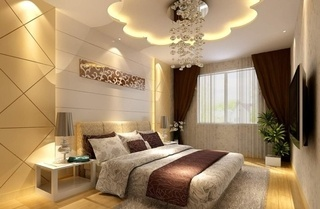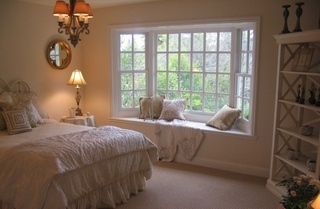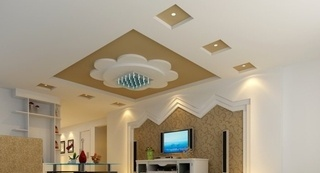|

# 集成吊顶与石膏吊顶的比较

目前家装的消费人群有两种，一种是改善居住环境的，另一种是结婚刚性需求的，而无论是哪一种，对于生活水平越来越高的今天，人们更注重的是装修时的环保性，和入住后的健康性。一、环保对比：

石膏顶很难达到个性效果与环保健康的完美结合，因为：只要是涂料就会有“毒素”——甲醛，而石膏吊顶不管做成什么样的，即使是最简单的白色效果，都需要在现场用涂料来完成最终的装饰效果，这是装修过程中的共识，所以石膏吊顶的环保健康就很难令人满意，如果你想做别的颜色，就要用到油漆，其“危害”就更大，其甲醛的含量指标更是令人担忧。

集成吊顶是以铝材为基材，铝本身就是一种可回收的环保金属，不含有毒物质，而且我们对铝本身的处理如表面材质色彩等，都是在出厂前已经完成，现场施工只是安装上的程序，不需要任何的辅助程序就能完成，完全能达到环保健康化的标准。

石膏板的安装废料不可回收，集成吊顶的安装下角料可以回收再利用。二、工期对比：

对于一心想尽快搬进新居的业主来说，施工工期也是他们重点考虑选材的因素，集成吊顶施工流程：顶部打丝杆——安装龙骨及配件——安装模块——完工，一般一户人家装一个客厅吊顶只需1-2天时间。

石膏板吊顶施工流程：顶部打丝杆——安装龙骨——吊板——刮腻子——一次涂料——二次涂料——完工，一般一户人家装一个石膏板客厅吊顶约需5-7天时间。其中最后三道流程是时间最长的，因为要等第一次涂料干了之后再刷第二次，所以在工期上要长很多。

三、个性化对比：

对于时下家装的主流消费人群——80/90后来说，一成不变的生活方式是不可想象的，对于装修风格也是一样，在家里的客厅中，我的家具能换、我的电器能换、我的软装能换、但是我的吊顶能换吗？

传统石膏吊顶是整体吊装后刷涂料，所以你不会把石膏吊顶敲掉重吊，那样会产生很大的灰尘和垃圾，很不环保；而且施工更会影响你的日常生活；所以吊了石膏吊顶一般都是用十年、十五年甚至更久而不能调整。只能任其一成不变地存在下去。

集成吊顶是一种组合式模块化的吊顶组合，卡扣式的安装方式，可方便您随时调整您的想法，且不影响您的日常生活，你想调整顶部模块色彩，可以，你想调整顶部的方形造型调整成圆形造型，可以，只要您要想法，就可改变你的吊顶形式。四、安全性能对比：

安全是家装最重要的一个衡量标准，所以受到业主们的特别重视，而防火安全是其中最主要的安全隐患。

石膏板本身具有防火性，但是用石膏板做的吊顶没有防火性，石膏板的支撑主要是靠两层纸，把纸烧掉以后板子就散了，容易造成顶部坍塌的危险。

集成吊顶的基材是铝质金属，其防火性能不言而喻。

五、持久耐用性对比：

持久耐用化是业主对于选择何种材料来装饰他们的客厅吊顶的主要因素，为什么呢？因为哪个业主也不希望刚装修好的房子，因为选材的问题而导致用了三五年就要重新调整，没有那么大的精力和时间。

石膏板吊顶在持久耐用方面表现如何呢？它是用涂料来掩盖安装时的拼缝，刚装修完成时，感觉效果可以，但是时间一长，如果工艺不到位，原来掩盖掉的拼缝和乳胶漆就会开裂，影响整体的美观度，这时你如果是保持美观，就要重新涂刷，这样就会影响你的日常生活。同时虽然石膏板本身具有很好的防潮性能，但是表面的涂料却不具备防潮功能，特别是对于南方的业主来说，更是头疼的问题，因为南方湿气大，特别是梅雨季节，整个屋内都是潮气，久而久之，用涂料刷白的石膏吊顶时间一长就会发霉黄变，您刚住了几年的房子就有可能黄变？您能忍受吗？而如果重新整治所需要的勇气，您能承受吗？集成吊顶是全模块化组合，虽然有缝，但是就像瓷砖一样，可以作为装饰线条来用，完全不影响整体效果。更不会出现开裂的现象，而经过表面处理过的铝板，颜色能持久如新，任何天气条件下，都能保证使用寿命在二十年以上。即使需要调整，只要换个模块就可轻松完成。

通过对比，相信大家不难看出集成吊顶在客厅吊顶中在风格与色彩上完全能够代替石膏吊顶，在功能上更能完的表现出来，远胜于石膏吊顶。当然这只是一组对比，石膏吊顶一般用白色乳胶漆做装饰，整体以一种白色为主，没有档次和品位，不能很好地与整体客厅效果协调，集成吊顶有上百款时下主流装饰色彩的模块，与不同风格的元素，能够很完美地与整体客厅效果协调，达到风格与色彩的完美统一，能够体现品位和档次。

`声明：本文由入驻焦点开放平台的作者撰写，除焦点官方账号外，观点仅代表作者本人，不代表焦点立场错误信息举报电话： 400-099-0099，邮箱：jubao@vip.sohu.com，或点此进行意见反馈，或点此进行举报投诉。`A B C D E F G H J K L M N P Q R S T W X Y Z
A - B - C - D - E
• A
• 鞍山
• 安庆
• 安阳
• 安顺
• 安康
• 澳门
• B
• 北京
• 保定
• 包头
• 巴彦淖尔
• 本溪
• 蚌埠
• 亳州
• 滨州
• 北海
• 百色
• 巴中
• 毕节
• 保山
• 宝鸡
• 白银
• 巴州
• C
• 承德
• 沧州
• 长治
• 赤峰
• 朝阳
• 长春
• 常州
• 滁州
• 池州
• 长沙
• 常德
• 郴州
• 潮州
• 崇左
• 重庆
• 成都
• 楚雄
• 昌都
• 慈溪
• 常熟
• D
• 大同
• 大连
• 丹东
• 大庆
• 东营
• 德州
• 东莞
• 德阳
• 达州
• 大理
• 德宏
• 定西
• 儋州
• 东平
• E
• 鄂尔多斯
• 鄂州
• 恩施
F - G - H - I - J
• F
• 抚顺
• 阜新
• 阜阳
• 福州
• 抚州
• 佛山
• 防城港
• G
• 赣州
• 广州
• 桂林
• 贵港
• 广元
• 广安
• 贵阳
• 固原
• H
• 邯郸
• 衡水
• 呼和浩特
• 呼伦贝尔
• 葫芦岛
• 哈尔滨
• 黑河
• 淮安
• 杭州
• 湖州
• 合肥
• 淮南
• 淮北
• 黄山
• 菏泽
• 鹤壁
• 黄石
• 黄冈
• 衡阳
• 怀化
• 惠州
• 河源
• 贺州
• 河池
• 海口
• 红河
• 汉中
• 海东
• 怀来
• I
• J
• 晋中
• 锦州
• 吉林
• 鸡西
• 佳木斯
• 嘉兴
• 金华
• 景德镇
• 九江
• 吉安
• 济南
• 济宁
• 焦作
• 荆门
• 荆州
• 江门
• 揭阳
• 金昌
• 酒泉
• 嘉峪关
K - L - M - N - P
• K
• 开封
• 昆明
• 昆山
• L
• 廊坊
• 临汾
• 辽阳
• 连云港
• 丽水
• 六安
• 龙岩
• 莱芜
• 临沂
• 聊城
• 洛阳
• 漯河
• 娄底
• 柳州
• 来宾
• 泸州
• 乐山
• 六盘水
• 丽江
• 临沧
• 拉萨
• 林芝
• 兰州
• 陇南
• M
• 牡丹江
• 马鞍山
• 茂名
• 梅州
• 绵阳
• 眉山
• N
• 南京
• 南通
• 宁波
• 南平
• 宁德
• 南昌
• 南阳
• 南宁
• 内江
• 南充
• P
• 盘锦
• 莆田
• 平顶山
• 濮阳
• 攀枝花
• 普洱
• 平凉
Q - R - S - T - W
• Q
• 秦皇岛
• 齐齐哈尔
• 衢州
• 泉州
• 青岛
• 清远
• 钦州
• 黔南
• 曲靖
• 庆阳
• R
• 日照
• 日喀则
• S
• 石家庄
• 沈阳
• 双鸭山
• 绥化
• 上海
• 苏州
• 宿迁
• 绍兴
• 宿州
• 三明
• 上饶
• 三门峡
• 商丘
• 十堰
• 随州
• 邵阳
• 韶关
• 深圳
• 汕头
• 汕尾
• 三亚
• 三沙
• 遂宁
• 山南
• 商洛
• 石嘴山
• T
• 天津
• 唐山
• 太原
• 通辽
• 铁岭
• 泰州
• 台州
• 铜陵
• 泰安
• 铜仁
• 铜川
• 天水
• 天门
• W
• 乌海
• 乌兰察布
• 无锡
• 温州
• 芜湖
• 潍坊
• 威海
• 武汉
• 梧州
• 渭南
• 武威
• 吴忠
• 乌鲁木齐
X - Y - Z
• X
• 邢台
• 徐州
• 宣城
• 厦门
• 新乡
• 许昌
• 信阳
• 襄阳
• 孝感
• 咸宁
• 湘潭
• 湘西
• 西双版纳
• 西安
• 咸阳
• 西宁
• 仙桃
• 西昌
• Y
• 运城
• 营口
• 盐城
• 扬州
• 鹰潭
• 宜春
• 烟台
• 宜昌
• 岳阳
• 益阳
• 永州
• 阳江
• 云浮
• 玉林
• 宜宾
• 雅安
• 玉溪
• 延安
• 榆林
• 银川
• Z
• 张家口
• 镇江
• 舟山
• 漳州
• 淄博
• 枣庄
• 郑州
• 周口
• 驻马店
• 株洲
• 张家界
• 珠海
• 湛江
• 肇庆
• 中山
• 自贡
• 资阳
• 遵义
• 昭通
• 张掖
• 中卫

1室1厅1厨1卫1阳台

1
2
3
4
5

0
1
2

1

1

0
1
2
3报名成功，资料已提交审核A B C D E F G H J K L M N P Q R S T W X Y Z
A - B - C - D - E
• A
• 鞍山
• 安庆
• 安阳
• 安顺
• 安康
• 澳门
• B
• 北京
• 保定
• 包头
• 巴彦淖尔
• 本溪
• 蚌埠
• 亳州
• 滨州
• 北海
• 百色
• 巴中
• 毕节
• 保山
• 宝鸡
• 白银
• 巴州
• C
• 承德
• 沧州
• 长治
• 赤峰
• 朝阳
• 长春
• 常州
• 滁州
• 池州
• 长沙
• 常德
• 郴州
• 潮州
• 崇左
• 重庆
• 成都
• 楚雄
• 昌都
• 慈溪
• 常熟
• D
• 大同
• 大连
• 丹东
• 大庆
• 东营
• 德州
• 东莞
• 德阳
• 达州
• 大理
• 德宏
• 定西
• 儋州
• 东平
• E
• 鄂尔多斯
• 鄂州
• 恩施
F - G - H - I - J
• F
• 抚顺
• 阜新
• 阜阳
• 福州
• 抚州
• 佛山
• 防城港
• G
• 赣州
• 广州
• 桂林
• 贵港
• 广元
• 广安
• 贵阳
• 固原
• H
• 邯郸
• 衡水
• 呼和浩特
• 呼伦贝尔
• 葫芦岛
• 哈尔滨
• 黑河
• 淮安
• 杭州
• 湖州
• 合肥
• 淮南
• 淮北
• 黄山
• 菏泽
• 鹤壁
• 黄石
• 黄冈
• 衡阳
• 怀化
• 惠州
• 河源
• 贺州
• 河池
• 海口
• 红河
• 汉中
• 海东
• 怀来
• I
• J
• 晋中
• 锦州
• 吉林
• 鸡西
• 佳木斯
• 嘉兴
• 金华
• 景德镇
• 九江
• 吉安
• 济南
• 济宁
• 焦作
• 荆门
• 荆州
• 江门
• 揭阳
• 金昌
• 酒泉
• 嘉峪关
K - L - M - N - P
• K
• 开封
• 昆明
• 昆山
• L
• 廊坊
• 临汾
• 辽阳
• 连云港
• 丽水
• 六安
• 龙岩
• 莱芜
• 临沂
• 聊城
• 洛阳
• 漯河
• 娄底
• 柳州
• 来宾
• 泸州
• 乐山
• 六盘水
• 丽江
• 临沧
• 拉萨
• 林芝
• 兰州
• 陇南
• M
• 牡丹江
• 马鞍山
• 茂名
• 梅州
• 绵阳
• 眉山
• N
• 南京
• 南通
• 宁波
• 南平
• 宁德
• 南昌
• 南阳
• 南宁
• 内江
• 南充
• P
• 盘锦
• 莆田
• 平顶山
• 濮阳
• 攀枝花
• 普洱
• 平凉
Q - R - S - T - W
• Q
• 秦皇岛
• 齐齐哈尔
• 衢州
• 泉州
• 青岛
• 清远
• 钦州
• 黔南
• 曲靖
• 庆阳
• R
• 日照
• 日喀则
• S
• 石家庄
• 沈阳
• 双鸭山
• 绥化
• 上海
• 苏州
• 宿迁
• 绍兴
• 宿州
• 三明
• 上饶
• 三门峡
• 商丘
• 十堰
• 随州
• 邵阳
• 韶关
• 深圳
• 汕头
• 汕尾
• 三亚
• 三沙
• 遂宁
• 山南
• 商洛
• 石嘴山
• T
• 天津
• 唐山
• 太原
• 通辽
• 铁岭
• 泰州
• 台州
• 铜陵
• 泰安
• 铜仁
• 铜川
• 天水
• 天门
• W
• 乌海
• 乌兰察布
• 无锡
• 温州
• 芜湖
• 潍坊
• 威海
• 武汉
• 梧州
• 渭南
• 武威
• 吴忠
• 乌鲁木齐
X - Y - Z
• X
• 邢台
• 徐州
• 宣城
• 厦门
• 新乡
• 许昌
• 信阳
• 襄阳
• 孝感
• 咸宁
• 湘潭
• 湘西
• 西双版纳
• 西安
• 咸阳
• 西宁
• 仙桃
• 西昌
• Y
• 运城
• 营口
• 盐城
• 扬州
• 鹰潭
• 宜春
• 烟台
• 宜昌
• 岳阳
• 益阳
• 永州
• 阳江
• 云浮
• 玉林
• 宜宾
• 雅安
• 玉溪
• 延安
• 榆林
• 银川
• Z
• 张家口
• 镇江
• 舟山
• 漳州
• 淄博
• 枣庄
• 郑州
• 周口
• 驻马店
• 株洲
• 张家界
• 珠海
• 湛江
• 肇庆
• 中山
• 自贡
• 资阳
• 遵义
• 昭通
• 张掖
• 中卫• 手机• 分享
• 设计
免费设计
• 计算器
装修计算器
• 入驻
合作入驻
• 联系
联系我们
• 置顶
返回顶部